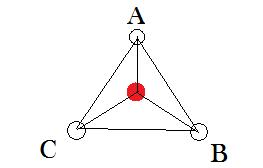#### You may also like### Lunar Leaper

Gravity on the Moon is about 1/6th that on the Earth. A pole-vaulter 2 metres tall can clear a 5 metres pole on the Earth. How high a pole could he clear on the Moon?### Which Twin Is Older?

A simplified account of special relativity and the twins paradox.### Whoosh

A ball whooshes down a slide and hits another ball which flies off the slide horizontally as a projectile. How far does it go?

##### Age 16 to 18Challenge Level

Clearly, a configuration of just 2 charged particles cannot remain stationary.

I then considered a configuration of 3 charges particles.

I considered whether an equilateral triangle of like charges, with an unlike charge in the centre, could be finely balanced in a plane. Of course any nudge outside of the plane would break the equilibrium. This has the potential to be stable: consider particle $A$ in the diagram; the horizontal force from particles $C$ and $B$ will cancel out leaving a net force in the positive vertical direction. By choosing the magnitude of the central force, we can cancel out this force.To see how this would work in detail, I resolved the forces at one vertex of the triangle, in the direction the vertex is pointing, and letting the side length of the equilateral triangle be $d$, and the vertex charge be $q_v$, and the central charge be $q_c$, I could say that
$$F_A = 0 = 2\frac{kq_vq_v}{d^2}\cos30 - \frac{kq_cq_v}{\left(\frac{d}{\sqrt{3}}\right)^2}$$

$\therefore q_v = \sqrt{3}q_c$ would satisfy this arrangement.

Seeing this, I wondered if it might be possible to find a general result for a regular polygon with a central unlike charge (perhaps $q_v = \sqrt{\mbox{polygon order}}q_c$?)

But resolving the same way on a square, we find that
$$F_A = 0 = 2\frac{kq_vq_v}{d^2}\cos45 + \frac{kq_vq_v}{\left(\sqrt{2}d\right)^2} - \frac{kq_cq_v}{\left(\frac{d}{\sqrt{2}}\right)^2} \rightarrow q_c = \frac{2\sqrt{2} + 1}{4}q_v \rightarrow$$
no obvious correlation between the order of the polygon and the required relationship between the charge for equilibrium, in fact the direction of the trend is the opposite. $q_v \approx 1.73q_c$ for a triangle and $q_v \approx 1.045q_c$ for a square.

I am reasonably convinced by this that it would be possible to find a stable choice of charges for any regular polygon with an unlike charge in the centre.

I am also sure it would work for a regular polyhedron.

It is not possible to have an arrangement such that only one charge moves due to Newton 3. It is possible that only 2 move, for example three like charges equally spaced

If one were fixed, of course it would be possible that just one could move.

As mentioned, the planar configurations above are not stable. But what about a 3-D configuration, such as a tetrahedron with an unlike charge in the centre?

I wondered if a tetrahedral arrangement would be stable, and decide to find the appropriate charges, and then mathematically move the central charge by an amount $\delta$, to see if the result was enlightening.

Thinking about and drawing out a regular tetrahedron of edge length $l$, I can see that it can be made from 3 triangular pyramids. I calculated the dimensions by looking at a cross-section of the tetrahedron and seeing a right-angled triangle of hypotenuse $l$, base $\frac{l}{\sqrt{3}}$, and of side (pyramid height + pyramid side edge length) (calling them $h + s$). From trig, we know this sum is $\sqrt{\frac{2}{3}}l$, so we have that equation and we also have the fact that $s^2 = h^2 + (\frac{l}{\sqrt{3}})^2$ from the pyramid itself. Combining these we can find that $s = \sqrt{\frac{3}{8}}l$ and $h = \frac{\sqrt{6}}{12}l$. The central vertices of these pyramids meet in the centre of the tetrahedron. This helped me to formulate the Coulomb's law equation, again resolving forces at a vertex of the shape, and again in the direction the vertex is pointing, $\frac{3q_vq_v}{l^2}\cos(\theta) = \frac{q_cq_v}{\left(\sqrt{\frac{3}{8}}l\right)^2}$, where $\cos(\theta) = h/s = 1/3 \therefore q_v = \frac{8}{3}q_c\;.$

Having done this, I was glad it was an interesting exercise in proving the earlier part of the question in 3D rather than simple planar arrangements, because I realised it probably wasn't necessary for the last part of the question. Because it looks quite simply that if you move the central charge some amount $\delta$ towards a vertex, that vertex will have a net attractive force, and the other vertices will have a net repulsive force, and so the arrangement will tend to fly apart. Although I did not reach a conclusive mathematical proof of this.

One possibility for a stable configuration might be to have unlike charges orbiting. We know from our own solar system that mathematically similar arrangments are not broken up by slight nudges.